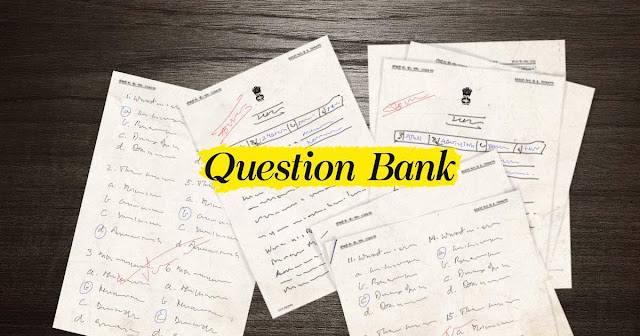Sheni Blog (www.shenischool.in): SRI SHARADAMBA HSS SHENI School Blog

Sheni Blog Plus Two Mathematics Question Paper | Sheni Blog 12th Mathematics Model Question Papers PDFSheni Blog Plus Two Mathematics Question Paper | Sheni Blog 12th Mathematics Model Question Papers PDF

Sheni Blog Plus Two Mathematics Question Paper: Here, we have listed down the Kerala Board important questions for Sheni Blog Plus Two Mathematics pdf for each chapter in the syllabus of Mathematics. Even solutions for every question have been provided. The topmost teachers of Mathematics have solved Kerala SCERT board Sheni Blog Plus Two Mathematics important questions chapter wise with solutions for you to make your Plus Two Mathematics exam preparation easier.

Sheni Blog Plus Two Mathematics Question Paper with Solutions PDF

Sheni Blog Plus Two Mathematics Question Paper with Solutions PDF which are available for free download, will not only probe your understanding of the subject matter but also prepare you to face various competitive exams which are held outside the borders of your classroom like the JEE, NEET, and common engineering entrance exams. If you’ve always struggled with finding question papers, then Sheni Blog is here with important questions for Sheni Blog Plus Two Mathematics Chapter Wise Question Papers Free PDF download will reveal areas you need to work on once you try and answer all the questions in the files.

 Board Kerala State Board Material Question Papers Type PDF/DOC Class Plus Two Subject Mathematics Provider Sheni Blog

1. Visit the website of Sheni Blog.
2. Look for Question Papers with Answers.
3. From the list of Sheni Blog Plus Two Question Papers that will be displayed.

Students can download the Sheni Blog Plus Two Mathematics Model Question Papers PDF from the link provided below:
• Plus Two Mathematics Chapter Wise Questions & Answers

• Preparation Tips for using Sheni Blog Plus Two Mathematics Question Papers

Hope you haven’t found any problem while downloading the pdf files above, if you do then please let us know by mentioning it in the comment box below.
So, here are some important tips, tricks, and suggestions which you should follow while preparing for mathematics for the board exams.
• If you will practice the Sheni Blog Plus Two Mathematics or Model papers then you will come to know that all the questions are from the SCERT books. The way of asking the question may be different, but no Two can say that the questions are out of the syllabus.
• Learn each and every formula, this is a must step, and you must learn each and every formula. You can get 15 extra marks just by remembering the formulas.
• Practice it, reading won’t help
• Practice at night time, during free time in school, and not in the morning. Use morning time to learn the formulas.
• We get sluggish in our free time, therefore, it is hard to practice other subjects like physics and chemistry as they can get boring, but Mathematics is a subject that will keep you busy.
• Find out your weak points and work hard on them, clear your doubts with your friends and teachers before it gets too late.
• This mistake costs a lot to most of the students, if you are facing problems regarding some question or topic then you should clear it right away. Now don’t tell me that you have no Two to ask because there is always someTwo that you can ask.

Do not let any sample and paper go. Solve them with full focus, this will make you predict the pattern of the board exams. Do you know that you can secure extra 20% marks just by solving the papers?

FAQs Regarding Sheni Blog Plus Two Mathematics Model Wise Question Papers

The FAQs Regarding Sheni Blog Plus Two Mathematics Model Question Papers and Answers are given below:

What is the advantage of solving Sheni Blog Plus Two Mathematics Model Question Papers?

Solving Sheni Blog Plus Two Mathematics question papers will help students in terms of understanding the Paper Pattern and section-wise distribution of marks.

Is SCERT enough for Sheni Blog Plus Two Mathematics Exams?

Yes, SCERT is more than enough for Sheni Blog Plus Two Kerala board exams since all the questions will be prepared based on the SCERT Sheni Blog Plus Two Mathematics book.